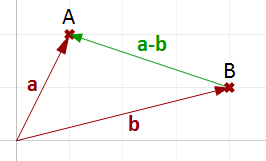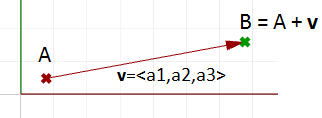Vectors in Python

## Vectors

Similar to 3D points, 3D vectors are stored as Vector3d structures. They can be thought as a zero-based, one-dimensional list that contain three numbers. These three number represent to the X, Y and Z coordinate direction of the vector.

vector3d contains [1.0, 2.0, 3.0]


Here is an easy way to construct a vector:

import rhinoscriptsyntax as rs

vec = rs.VectorCreate(1.0, 2.0, 3.0)


A Vector3d’s coordinates can be accessed as a list, one element at a time:

import rhinoscriptsyntax as rs

vec = rs.VectorCreate(1.0, 2.0, 3.0)

print(vec) #Prints the X coordinate of the Vector3d
print(vec) #Print the Y coordinate of the Vector3d
print(vec) #Print the Z coordinate of the Vector3d


The coordinates of a Vector3d may also be accessed through its .X, .Y and .Z properties:

import rhinoscriptsyntax as rs

vec = rs.VectorCreate(1.0, 2.0, 3.0)

print(vec.X) # Prints the X coordinate of the Vector3d
print(vec.Y) # Print the Y coordinate of the Vector3d
print(vec.Z) # Print the Z coordinate of the Vector3d


To change the individual coordinate of a Vector3d, simply assign a new value to the coordinate through the index location or coordinate property:

import rhinoscriptsyntax as rs

point1 = [1,2,3]
point2 = [4,6,7]
vec = rs.VectorCreate(1.0, 2.0, 3.0)

vec = 5.0 # Sets the X coordinate to 5.0
vec.Y = 45.0 # Sets the Y coordinate to 45.0

print(vec) #Print the new coordinates


To find the vector between two points, use vector subtraction:point1 = [1,2,3]
point2 = [4,5,6]

vec = point2 - point1

print(vec) #prints the new coordinates.


In the above example, the vector goes from point1 to point2. Reversing this direction is a common mistake. It is importatnt to be sure that the starting point is subtraced from the ending point.

Vectors can also be added to points to create new point locations. Here is an example of moving a point location by a vector:#  A base point
point1 = [1,1,1]

# A vector with a direction of 2 units in the X direction
vector1 = [2,0,0]

# Setting the coordinate of point1 to to units more in the X direction.
point1 = point1 + vector1
# point1 + vector1 = [1+2, 1+0, 1+0] = [3,1,1]


Use a for loop to walk through each coordinate in succession:

for c in vec:
print c # This will loop through each coordinate in the vector3d


RhinoScriptSyntax contains a number of methods to manipulate vectors. See RhinoScript Points and Vectors Methods for details.